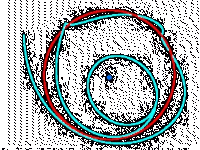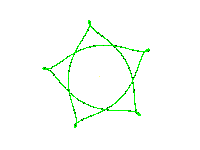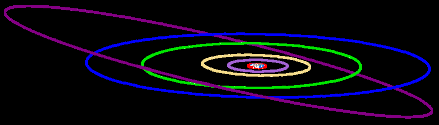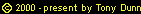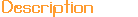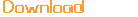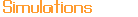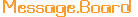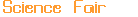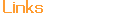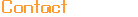### Period Calculator

An object's orbital period can be computed from its semi-major axis and the mass of the body it orbits using the following formula: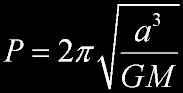a is the semi-major axis of the object P is the orbital period G is the gravitational constant M is the mass of the parent bodyDefault units Acceptable substitute units a meters (m) centimeters (cm), kilometers (km), miles (mi), feet (ft), Earth radii (Re), Solar radii (Rs), astronomical units (AU), light years (ly), parsec (pc) T seconds (s) minutes (m), hours (h), days (d), years (yr) G N m2kg-2 M kilograms (kg) grams (g), Earth masses (Me), Solar masses (Ms)

Sample problems:

1. A satellite orbits the Earth. It's semi-major axis is 7000 km. What is the satellite's orbital period in hours?
 Enter 7000 km for a and 1 Me for M. The calculator gives you an answer of 5830.318 s. Highlight the s and type h. It now gives you an answer of 1.6195 hr.
2. Astronomers discover a distant planet orbiting the Sun. It's semi-major axis is 1 light year. What is the planet's orbital period in years?
 Enter 1 LY for a and 1 Ms for M. The calculator gives you an answer of 501850205474964.06 s. Highlight the s and type y. It now gives you an answer of 15902421.421915896 yr.